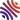## 33 publications1. Application of Padé approximation to Euler's constant and Stirling's formula ( and ), In Ramanujan J., volume 54, . [hal-lmpa]
2. Diagonal convergence of the remainder Padé approximants for the Hurwitz zeta function ( and ), In J. Number Theory, volume 222, . [hal-lmpa]
3. Remainder Pad'e approximants for hypergeometric series ( and ), In Integral Transforms Spec. Funct., volume 30, .
4. Remainder Pad'e approximants for the Hurwitz zeta function (), In Results Math., volume 74, .
5. Expansion of Euler's constant in terms of zeta numbers ( and ), In J. Math. Anal. Appl., volume 398, .
6. Series involving zeta and related functions (), In Appl. Math. Comput., volume 217, .
7. Recurrence for values of the zeta function (), In Appl. Numer. Math., volume 60, .
8. Padé approximation and Apostol-Bernoulli and Apostol-Euler polynomials (), In J. Comput. Appl. Math., volume 233, .
9. Legendre modified moments for Euler's constant (), In J. Comput. Appl. Math., volume 219, .
10. International conference on approximation and iterative methods (, and ), In J. Comput. Appl. Math., volume 219, .
11. Remainder Padé approximants for the exponential function ( and ), In Constr. Approx., volume 25, . [hal-lmpa]
12. Quantitative irrationality for sums of reciprocals of Fibonacci and Lucas numbers ( and ), In Ramanujan J., volume 11, .
13. Hermite-Padé approximations and Apéry's theorem ( and ), In Vestnik Moskov. Univ. Ser. I Mat. Mekh., .
14. Convergence acceleration of Gauss-Chebyshev quadrature formulae ( and ), Chapter in , volume 34, .
15. Approximation and prediction of the numerical solution of some Burgers problems ( and ), Chapter in , volume 33, .
16. Irrationality measures for the series of reciprocals from recurrence sequences ( and ), In J. Number Theory, volume 96, .
17. Diophantine approximations using Padé approximations (), Chapter in , volume 122, .
18. On totally monotonic families of sequences ( and ), In Numer. Math., volume 84, .
19. Partial Padé prediction ( and ), In Numer. Algorithms, volume 20, .
20. On the irrationality of $\sum t^n/(A\alpha^n+B\beta^n)$ (), In J. Number Theory, volume 73, .
21. An acceleration property of the $E$-algorithm for alternate sequences ( and ), In Adv. Comput. Math., volume 5, .
22. A new proof of the irrationality of $\zeta(2)$ and $\zeta(3)$ using Padé approximants (), In J. Comput. Appl. Math., volume 67, .
23. Rate of convergence of Padé approximants for a particular Wynn series (), In Appl. Numer. Math., volume 17, .
24. Acceleration of some logarithmic sequences (), In J. Comput. Appl. Math., volume 55, .
25. Acceleration property for the $E$-algorithm and an application to the summation of series (), In Adv. Comput. Math., volume 2, .
26. Dirac masses determination with orthogonal polynomials and $\epsilon$-algorithm. Application to totally monotonic sequences (), In J. Approx. Theory, volume 71, .
27. Acceleration property for the columns of the $E$-algorithm ( and ), In Numer. Algorithms, volume 2, .
28. Dirac masses detection in a density on $[-1,1]$ from its moments. Applications to singularities of a function (), Chapter in Orthogonal polynomials and their applications (Erice, 1990), Baltzer, Basel, volume 9, .
29. Approximation of weight function and approached Padé approximants (), Chapter in , volume 32, .
30. Determinantal expression for partial Padé approximants (), In Appl. Numer. Math., volume 6, .
31. Stieltjes- and Geronimus-type polynomials (), In J. Comput. Appl. Math., volume 21, .
32. Calculation of poles of meromorphic functions with q-d, r-s and $\epsilon$-algorithms. Acceleration of these processes (), In J. Comput. Appl. Math., volume 19, .
33. Padé-type approximants with orthogonal generating polynomials (), In J. Comput. Appl. Math., volume 9, .